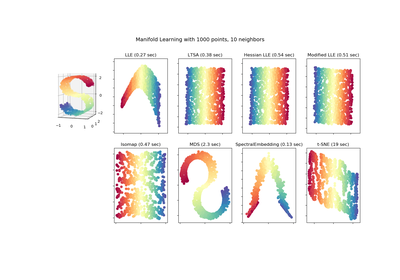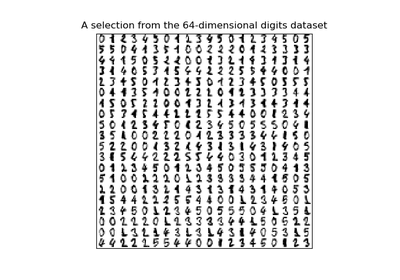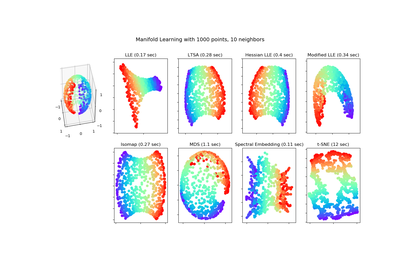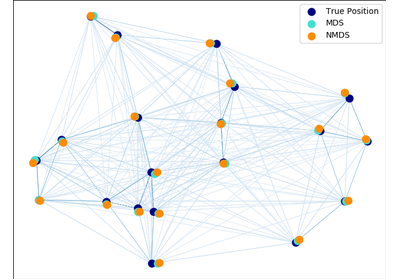# `sklearn.manifold`.MDS¶

class `sklearn.manifold.``MDS`(n_components=2, metric=True, n_init=4, max_iter=300, verbose=0, eps=0.001, n_jobs=1, random_state=None, dissimilarity=’euclidean’)[source]

Multidimensional scaling

Read more in the User Guide.

Parameters: n_components : int, optional, default: 2 Number of dimensions in which to immerse the dissimilarities. metric : boolean, optional, default: True If `True`, perform metric MDS; otherwise, perform nonmetric MDS. n_init : int, optional, default: 4 Number of times the SMACOF algorithm will be run with different initializations. The final results will be the best output of the runs, determined by the run with the smallest final stress. max_iter : int, optional, default: 300 Maximum number of iterations of the SMACOF algorithm for a single run. verbose : int, optional, default: 0 Level of verbosity. eps : float, optional, default: 1e-3 Relative tolerance with respect to stress at which to declare convergence. n_jobs : int, optional, default: 1 The number of jobs to use for the computation. If multiple initializations are used (`n_init`), each run of the algorithm is computed in parallel. If -1 all CPUs are used. If 1 is given, no parallel computing code is used at all, which is useful for debugging. For `n_jobs` below -1, (`n_cpus + 1 + n_jobs`) are used. Thus for `n_jobs = -2`, all CPUs but one are used. random_state : int, RandomState instance or None, optional, default: None The generator used to initialize the centers. If int, random_state is the seed used by the random number generator; If RandomState instance, random_state is the random number generator; If None, the random number generator is the RandomState instance used by np.random. dissimilarity : ‘euclidean’ | ‘precomputed’, optional, default: ‘euclidean’ Dissimilarity measure to use: ‘euclidean’: Pairwise Euclidean distances between points in the dataset. ‘precomputed’: Pre-computed dissimilarities are passed directly to `fit` and `fit_transform`. embedding_ : array-like, shape (n_components, n_samples) Stores the position of the dataset in the embedding space. stress_ : float The final value of the stress (sum of squared distance of the disparities and the distances for all constrained points).

References

“Modern Multidimensional Scaling - Theory and Applications” Borg, I.; Groenen P. Springer Series in Statistics (1997)

“Nonmetric multidimensional scaling: a numerical method” Kruskal, J. Psychometrika, 29 (1964)

“Multidimensional scaling by optimizing goodness of fit to a nonmetric hypothesis” Kruskal, J. Psychometrika, 29, (1964)

Methods

 `fit`(X[, y, init]) Computes the position of the points in the embedding space `fit_transform`(X[, y, init]) Fit the data from X, and returns the embedded coordinates `get_params`([deep]) Get parameters for this estimator. `set_params`(**params) Set the parameters of this estimator.
`__init__`(n_components=2, metric=True, n_init=4, max_iter=300, verbose=0, eps=0.001, n_jobs=1, random_state=None, dissimilarity=’euclidean’)[source]
`fit`(X, y=None, init=None)[source]

Computes the position of the points in the embedding space

Parameters: X : array, shape (n_samples, n_features) or (n_samples, n_samples) Input data. If `dissimilarity=='precomputed'`, the input should be the dissimilarity matrix. y: Ignored. : init : ndarray, shape (n_samples,), optional, default: None Starting configuration of the embedding to initialize the SMACOF algorithm. By default, the algorithm is initialized with a randomly chosen array.
`fit_transform`(X, y=None, init=None)[source]

Fit the data from X, and returns the embedded coordinates

Parameters: X : array, shape (n_samples, n_features) or (n_samples, n_samples) Input data. If `dissimilarity=='precomputed'`, the input should be the dissimilarity matrix. y: Ignored. : init : ndarray, shape (n_samples,), optional, default: None Starting configuration of the embedding to initialize the SMACOF algorithm. By default, the algorithm is initialized with a randomly chosen array.
`get_params`(deep=True)[source]

Get parameters for this estimator.

Parameters: deep : boolean, optional If True, will return the parameters for this estimator and contained subobjects that are estimators. params : mapping of string to any Parameter names mapped to their values.
`set_params`(**params)[source]

Set the parameters of this estimator.

The method works on simple estimators as well as on nested objects (such as pipelines). The latter have parameters of the form `<component>__<parameter>` so that it’s possible to update each component of a nested object.

Returns: self :

## Examples using `sklearn.manifold.MDS`¶Comparison of Manifold Learning methodsManifold learning on handwritten digits: Locally Linear Embedding, Isomap…Manifold Learning methods on a severed sphereMulti-dimensional scaling